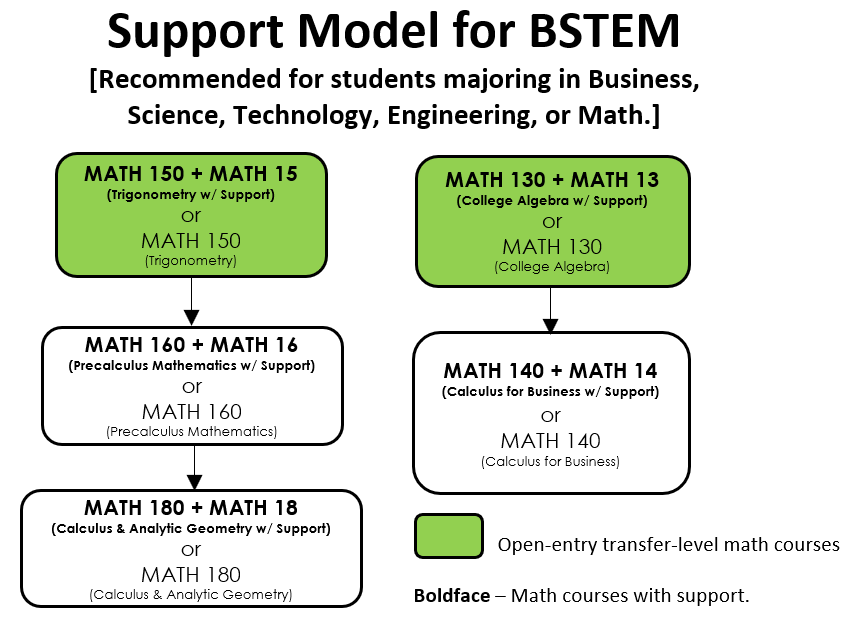# Math Corequisite CoursesMath corequisites are support courses designed to supplement topics needed for success in the paired course. Click below to get additional details about each corequisite course:

• Math 5 - Support Topics for Elementary Algebra
2.0 Units (Not Degree Applicable)
Pass/No Pass

Support will focus on essential arithmetic, algebraic, and geometric skills needed for success in Elementary Algebra. Course is for students concurrently enrolled in Math 51. Support topics include order of operations, rules of exponents, geometry, linear, quadratic, rational, and radical equations, systems of equations, basic graphing techniques, factoring, polynomial, rational, and radical expressions, and applications.

• Math 7 - Support Topics for Intermediate Algebra
2.0 Units (Not Degree Applicable)
Pass/No Pass

Support will focus on essential algebra skills needed for success in Intermediate Algebra. Course is for students concurrently enrolled in Math 71. Support topics include real numbers and their properties, algebraic expressions, integer and rational exponents, linear, quadratic, rational, radical, exponential, and logarithmic equations, systems of linear and nonlinear equations, absolute value equations and inequalities, graphing techniques, writing equations of lines, functions and relations, complex fractions, polynomial, rational, radical, and logarithmic expressions, and applications.

• Math 11 - Support Topics for Statistics
2.0 Units (Not Degree Applicable)
Pass/No Pass

Support will focus on essential skills required for success in Elementary Statistics. For students concurrently enrolled in Elementary Statistics (Math 110). Review and support topics include the use of calculators and technology to enhance understanding. Topics include inequality relationships between numbers, graphing and interpreting linear functions, applications with probabilities including notation, simulations, rounding, and significant digits. Applications with estimation, hypothesis testing using appropriate calculations, and conclusions.

• Math 13 - Support Topics for College Algebra
2.0 Units (Not Degree Applicable)
Pass/No Pass

Support will focus on essential algebra skills needed for success in College Algebra. Course is for students concurrently enrolled in Math 130. Support topics include algebraic expressions, integer and rational exponents, linear and nonlinear equations and inequalities, factoring polynomials, systems of equations and inequalities, graphing techniques and curve sketching, equations of lines, functions and relations, complex fractions, polynomial, rational, radical, exponential, and logarithmic expressions and equations, expanding binomials, and applications.

• Math 14 - Support Topics for Business Calculus
2.0 Units (Not Degree Applicable)
Pass/No Pass

Support will focus on essential algebra skills needed for success in Business Calculus. For students concurrently enrolled in Math 140. Algebra support topics include analyzing linear, polynomial, rational, radical, exponential, and logarithmic functions, solving various equations including polynomial, rational, radical, exponential, and logarithmic equations, solving various inequalities including polynomial, rational, and absolute value inequalities, graphing techniques and curve sketching, summation notation and Riemann sums, limits, differentiation rules and the chain rule, integration techniques, functions of several variables, double integration, and applications.

• Math 15 - Support Topics for Trigonometry
2.0 Units (Not Degree Applicable)
Pass/No Pass

Support will focus on essential geometry and algebra skills needed for success in Trigonometry. Course is for students concurrently enrolled in Math 150. Support topics include angle, triangle and parallelogram relationships, arc length and area of circles and sectors, linear transformations, simplifying algebraic expressions, solving algebraic equations, functions, graphs, unit conversions, and algebraic proofs.

• Math 16 - Support Topics for Precalculus
2.0 Units (Not Degree Applicable)
Pass/No Pass

Support will focus on essential trigonometry and algebra skills needed for success in Precalculus Mathematics. Course is for students concurrently enrolled in Math 160. Support topics include set notation, complex rational expressions and radical expressions, lines, piecewise-defined functions, circles, modeling with functions, factoring, asymptotes, solving inequalities, simplifying exponential expressions, evaluating trigonometric and inverse trigonometric functions, solving exponential, logarithmic, and trigonometric equations, vectors, and systems of equations.

• Math 18 - Support Topics for Calculus
2.0 Units (Not Degree Applicable)
Pass/No Pass

Support will focus on essential precalculus skills needed for success in Calculus. Course is for students concurrently enrolled in Calculus and Analytic Geometry (Math 180). Precalculus review topics include basic functions and transformations, equations of lines, difference quotients, asymptotes, absolute value inequalities, zeros of polynomial functions, function composition, applications of geometry and Pythagorean theorem, areas under curves using geometric formulas, sequences and series.

• Resources

• ######